# 1. 从细菌的趋化性谈起

## 0x1：物质化学浓度梯度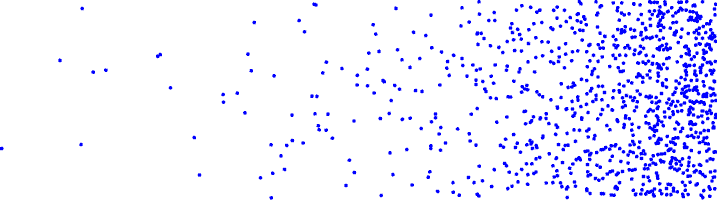## 0x2：趋化性细菌的运动方式

Engelmann 和 Pfeffer 发现细菌的运动不是任意的，而是定向移动。直到1960年，Alder深入研究了细菌趋化性的分子机制，提出大肠杆菌(Escherichia coli)对氨基酸以及糖的趋化性是由位于细胞表面的受体蛋白调节的，并由细胞内分子传递信号最终影响细菌的运动。

### 1. 顺时针旋转(clockwise，CW) - 按照本轮计算的优化方向进行迭代调整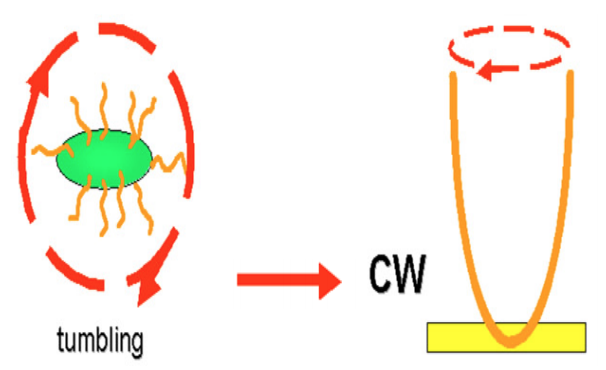### 2. 逆时针旋转(counter-clockwise， CCW)

#### 1）在存在局部浓度梯度变化的环境中调整方式 - GD过程

CCW过程中前进的这段，我们可以理解为梯度下降中，每次调整的总距离，即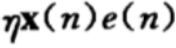1. 当诱导剂浓度降低或趋避剂浓度增加时，翻滚频率增加（缩短学习率），远离不利环境；
2. 反之，翻滚频率降低，细菌泳动，趋向有利环境（类似梯度下降中加入momentum动量因素，如果方向对了就继续保持）；

## 0x3：细菌趋化性分子机制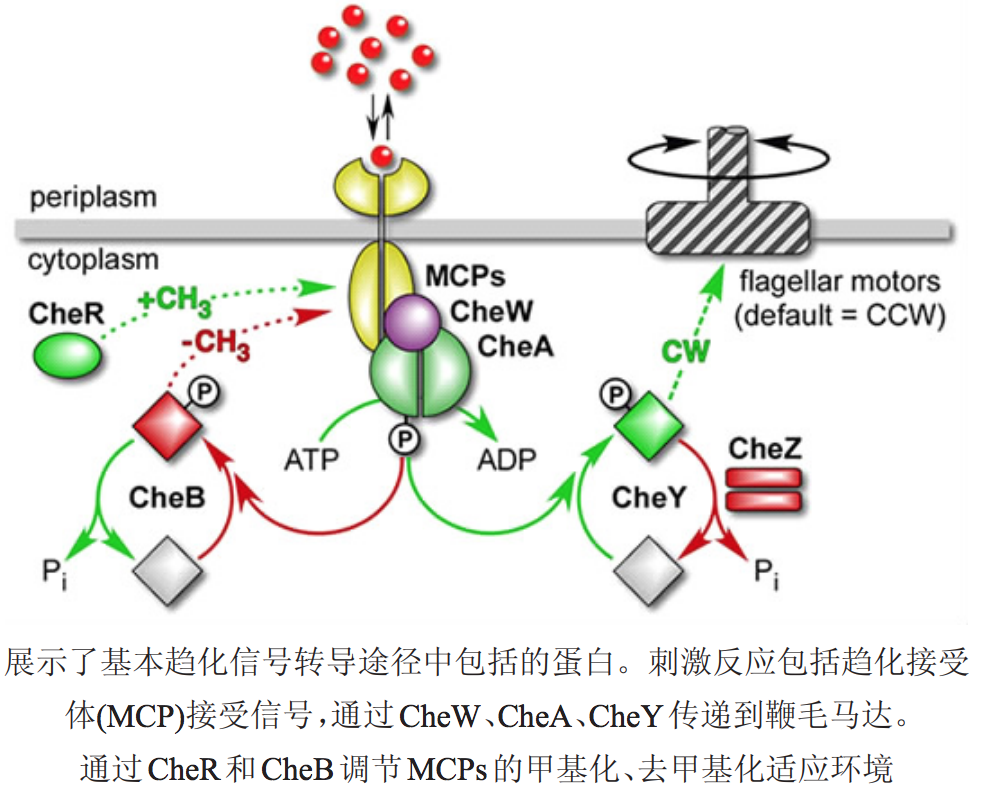1. 膜上趋化受体接收信号；
2. 从膜 受体到鞭毛马达的信号转导；
3. 对最初信号输入的适应。

http://www.casb.org.cn/PublishRoot/casb/2015/6/casb14110008.pdf

# 2. 随机游走数学模型## 0x1：一维空间下的等概论随机运动（伯努利随机游走）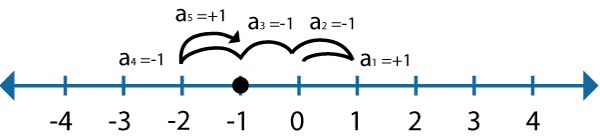### 1. 黑点随机运动的本质是什么？

1. 设函数 F(x) 为每次移动远离原点的距离（只针对这次移动而言，不考虑前一次的位置），这是一个随机变量函数；
2. 同时，F(x) 由代表每次移动的随机变量 an 决定，F(x) = F(an) = an。显然，an 是一个离散型随机变量，取值可能为 -1/+1，所以 F(x) 的可能取值也为 -1/+1；
3. F(x) 的损失函数 loss(E(x)) = loss（F(x) - 0）= loss（an - 0），代表了对黑点随机运动的一种抽象化数值考量；

### 1. 在均值损失函数背景下，对黑点随机运动的计算本质上是在计算最后黑点停留的位置(相对原点)

<d> = <(a1 + a2 + a3 + ... + aN)> = <a1> + <a2> + <a3> + ... + <aN>

<d> = <a1> + <a2> + <a3> + ... + <aN> = 0 + 0 + 0 + ... + 0 = 0

#### 2）黑点最后停止的位置等于黑点随机运动的数学期望

E(x) = -1 * 0.5 + 1 * 0.5 = 0

### 2. 在均方根(root-mean-squared)损失函数的背景下，对黑点随机运动的计算本质上是在计算黑点总共运行的距离(恒正值)

#### 1）计算均方根损失

<d2> = <(a1 + a2 + a3 + ... + aN)2> = <(a1 + a2 + a3 + ... + aN(a1 + a2 + a3 + ... + aN)>

= (<a12> + <a22> + <a32> + ... + <aN2>) + 2 (<a1a2> + <a1a3> + ... <a1aN> + <a2a3> + ... <a2aN> + ...)

<a12> = 1，因为a1取-1和+1的概率是相等的。同样的道理<a22>, <a32>, ...<aN2>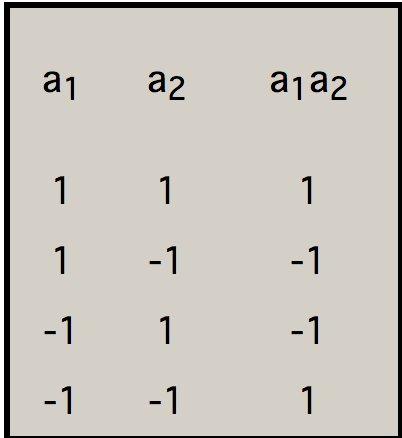<d2> = (<a12> + <a22> + <a32> + ... + <aN2>) + 2 (<a1a2> + <a1a3> + ... <a1aN> + <a2a3> + ... <a2aN> + ...)

= (1 + 1 + 1 + ... +1) + 2 (0 + 0 + ... + 0 + 0 + ...) = N

sqrt(<d2>) = sqrt(N)

## 0x3：Ramdom Walk在现实生活中的例子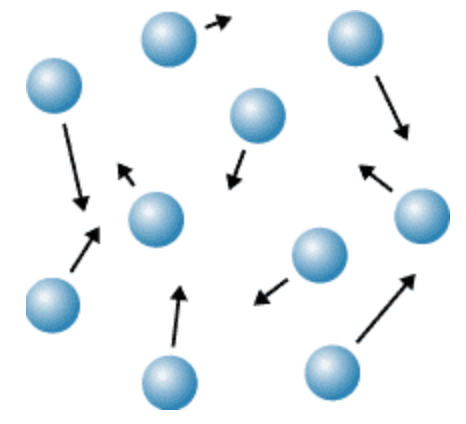## 0x4：包含偏置的随机游走(biased random walk)

1. 向不同方向行走的概率不相等，例如可能是 40%向左- 60%向右；
2. 向不同方向移动的步数(step size)不同，例如可能向左移动是1步，而向右是2步；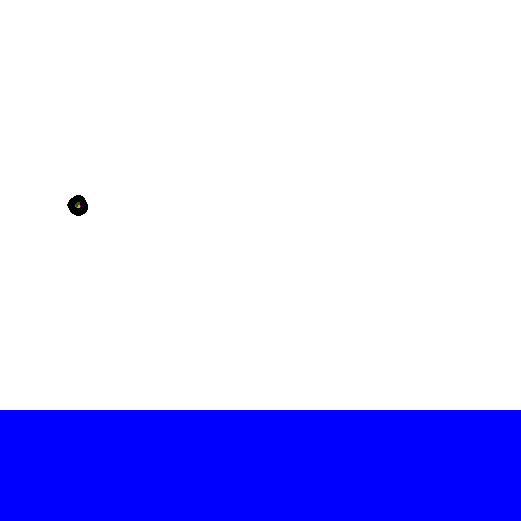## 0x5：细菌的趋化性本质上是一种偏置随机游走

### 2. 在有浓度梯度的环境下细菌呈现偏置随机游走

https://wenku.baidu.com/view/81d7a657a36925c52cc58bd63186bceb19e8edc2.html
http://www.mit.edu/~kardar/teaching/projects/chemotaxis%28AndreaSchmidt%29/random.htm
https://blog.csdn.net/u012655441/article/details/62216304

# 3. 细菌觅食优化算法 - Bacterial foraging optimization algorithm(BFOA)

## 0x1：BFOA的发展历史

1. 细菌以一种可以最大限度的提高单位时间内获得能量的方式去寻找食物；
2. 个体细菌通过发送信号来与其他的细菌进行交流；

BFOA的关键思想是在问题搜索空间模仿细菌趋化运动

## 0x2：BFOA算法的生物动力模型

### 1. 细菌系统中主要4种机制

#### 1）趋化运动

1. 翻转：当它们顺时针方向翻转时，每一根鞭毛都会拉动细胞。这导致了鞭毛的独立运动，并且最终以最少的代价去翻转。在糟糕的地方则频繁地翻转，去寻找一种营养梯度；
2. 游泳：逆时针方向移动鞭毛有助于细菌以非常快的速度游泳；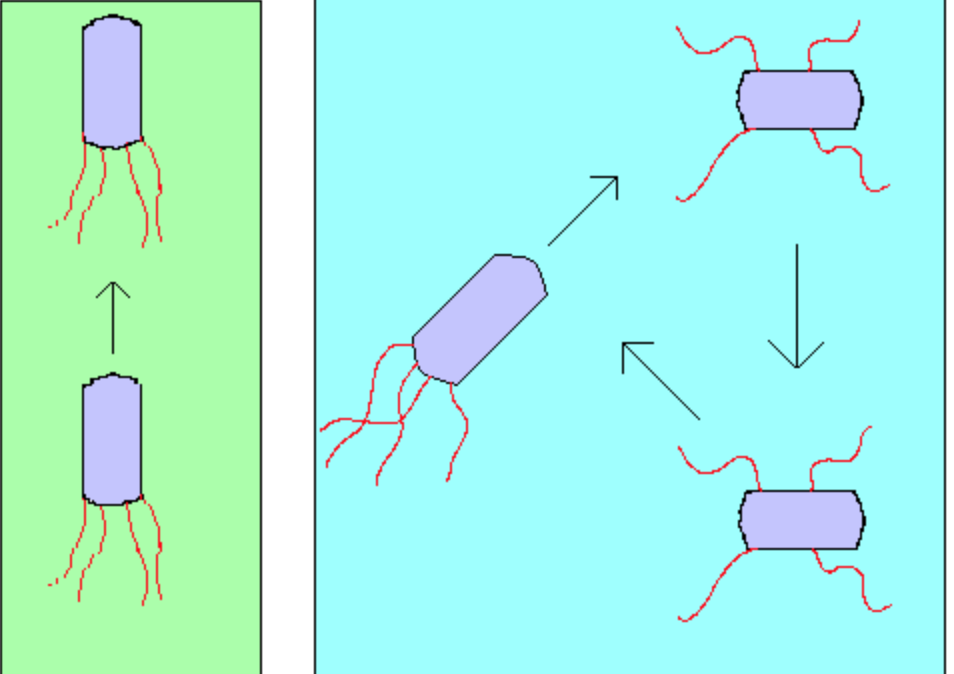Fig.1. Swim and tumble of a bacterium

### 2. 形式化定义细菌系统机制

BFOA模拟了细菌系统中的四个观察到的主要机制：趋化，群体，复制以及消除-分散。

BFOA解决的是一个无梯度优化问题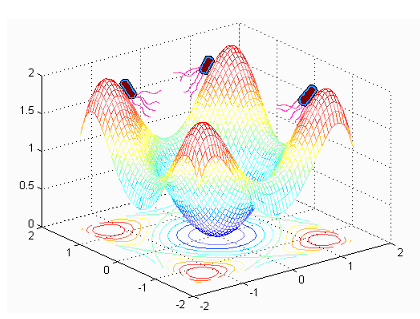Fig.2. 每个细菌都在寻找全局最优解，同时又一起构成一个整体群体

1. 定义 j 作为趋化步骤索引、k 作为复制步骤索引、l 作为消除-分散步骤索引；
2. p：搜索空间维度；
3. S：群体中细菌的总数；
4. Nc：趋化步骤的步数；
5. Ns：游泳长度；
6. Nre：复制步骤的次数；
7. Ned：消除-分散事件的次数；
8. Ped：消除-分散概率；
9. P( j , k , l ) = {θ ( j , k , l ) | i = 1,2,..., S}  ：代表 j 趋化步骤中，S细菌群体中的每一个成员的位置、k 复制步骤、l 消除-分散事件；
10. J( i，j，k，l )：表示在 i 细菌搜索定位中的消耗（代价）。我们可以将J当作“cost”代价来做参考（用优化理论的术语）；

#### 1）趋化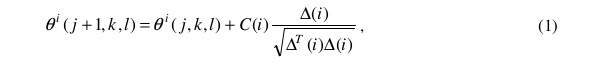#### 2）群聚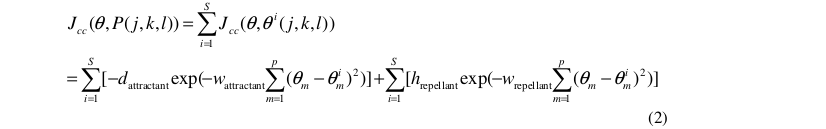S是细菌的总数；

p是将要被优化的变量的数量（即搜索空间的维度）；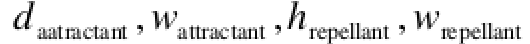是需要被搜索优化的系数（超参数）。

#### 4）消除和扩散

BFOA对这一现象的模式方式如下：

## 0x3：BFOA算法流程

【步骤 1】初始化参数 p，S，Nc，Ns，Nre，Ned，Ped，C(i)(i=1,2,…S)，θi

【步骤 2】消除-扩散 循环 loop：l=l+1
【步骤 3】复制循环 Loop：k=k+1
【步骤 4】趋化循环 Loop：j=j+1
[a] i=1,2,……S 细菌 i 进化趋化移动

[b] 计算适应值函数 J（i，j，k，l）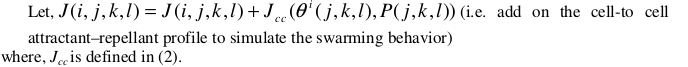[c] 让保存值，因为通过移动我们可能找到更好的。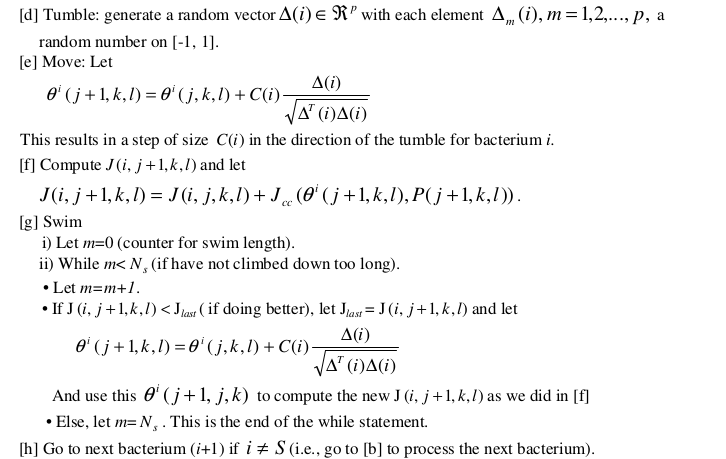【步骤 5】如果 j < Nc，跳转到步骤4。这种情况下继续进行趋化，因为细菌的生命并未结束。
【步骤 6】复制 - 消除：
[a] 根据给定的 k 跟 l，对i=1,2,……S,循环，让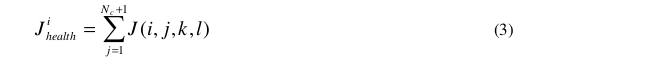[b] 拥有最高的 Jhealth值的 Sr 细菌死亡，拥有最低的Jhealth值的细菌分裂开来（结果放置在它们原先的父母的位置）。
【步骤 7】如果 K < Nre，跳到步骤3。这种情况下，我们没有达到制定复制的数量，所以，我们开始下一次趋化循环，产生后代。
【步骤 8】驱散：以概率Ped 进行循环 i=1,2,……S，驱散每一个细菌（保持持续不变的细菌种群数量）。如果 l < Ned，然后跳转到步骤2；否则结束。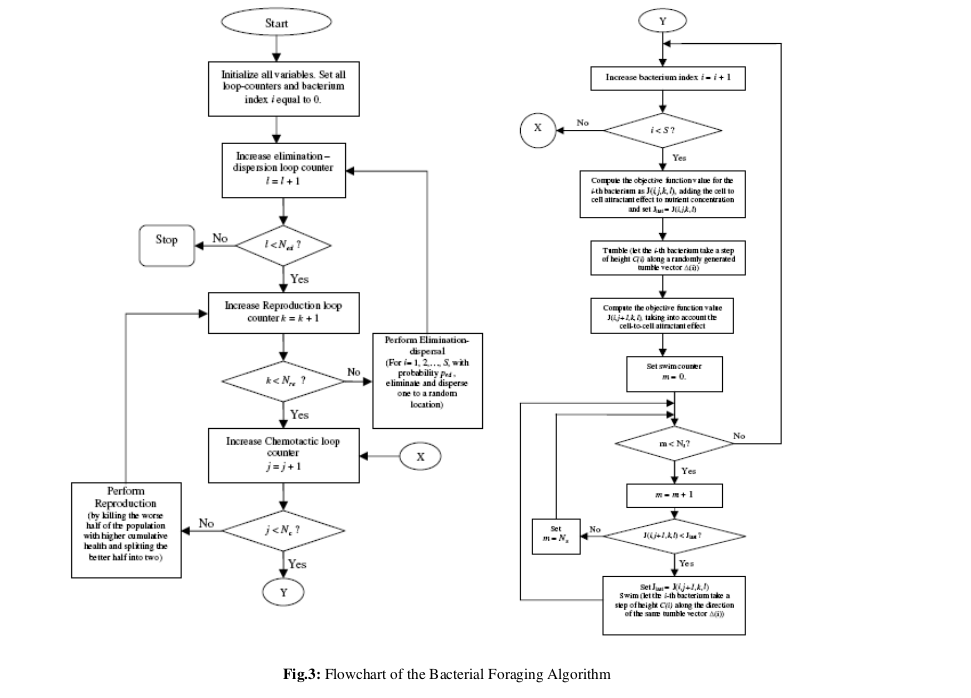http://www2.ece.ohio-state.edu/~passino/PapersToPost/BFO-IJSIR.pdf
https://ieeexplore.ieee.org/document/1004010
https://www.cnblogs.com/L-Arikes/p/3734353.html

# 4. 一种全局最优化算法  - 随机游走算法(Random Walk)

## 0x1：随机游走算法步骤

1）给定初始迭代点 x，初始行走步长λ，控制精度ϵ(ϵ是一个非常小的正数，用于控制结束算法)；

2）给定迭代控制次数 N，k 为当前迭代次数，置 k=1；

3）当 k < N 时，随机生成一个(−1,1) 之间的 n 维向量 u=(u1,u2,⋯,un)，(−1 < ui < 1，i=1,2,⋯,n)，并将其标准化得到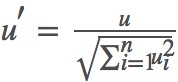。令 x1 = x + λu′，完成第一步游走尝试（注意这一步仅仅只是尝试）。

4）计算函数值

4.1）如果 f(x1) < f(x)，即找到了一个比初始值好的点，那么 k 重新置为1，同时将 x1 变为 x（完成实际的前进），回到第2步；

4.2）否则 k = k+1，回到第3步，继续其他方向的随机游走的尝试。

5）如果连续 N 次都找不到更优的值，则认为，最优解就在以当前最优解为中心，当前步长为半径的 n 维球内(如果是三维，则刚好是空间中的球体)。此时：

5.1）如果 λ < ϵ，则结束算法，即算法已经收敛；

5.2）否则，令 λ= λ / 2，减小学习率，回到第1步，开始新一轮游走。

## 0x2：随机游走的代码实现

#!/usr/bin/env python
# -*- coding: utf-8 -*-

from __future__  import print_function
import math
import random

N = 100     # 迭代次数
step = 0.5  # 初始步长
epsilon = 0.00001   # 收敛阈值
variables = 2   # 变量数目，搜索空间的维度
x = [49, 49]     # 初始点坐标
walk_num = 1    # 初始化随机游走次数

print("迭代次数:", N)
print("初始步长:", step)
print("epsilon:", epsilon)
print("变量数目:", variables)
print("初始点坐标:", x)

# 定义目标函数
def obj_function(p):
r = math.sqrt((p-50)**2 + (p-50)**2) + math.e
f = math.sin(r) / r + 1
return -f

# 开始随机游走
while(step > epsilon):  # 判断是否收敛停止算法
k = 1   # 初始化计数器
while(k < N):
u = [random.uniform(-1, 1) for i in range(variables)]       # 随机向量
# u1 为标准化之后的随机向量
u1 = [u[i] / math.sqrt(sum([u[i]**2 for i in range(variables)])) for i in range(variables)]
x1 = [x[i] + step*u1[i] for i in range(variables)]      # 随机游走尝试
if(obj_function(x1) < obj_function(x)):     # 如果找到了更优点
k = 1   # 重置 k
x = x1  # 更新坐标
else:
k += 1  # 继续下一次随机游走尝试
step = step/2
print("第 %d 次随机游走完成。" % walk_num)
walk_num += 1
print("随机游走次数:", walk_num-1)
print("最终最优点:", x)
print("最终最优值:", obj_function(x))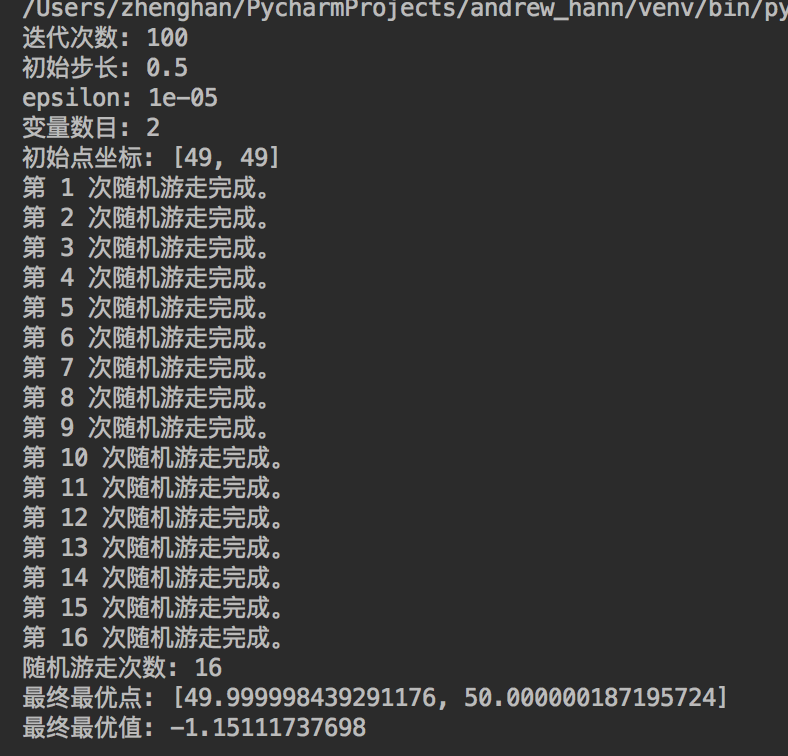### 1. 随机游走依然容易陷入局部鞍点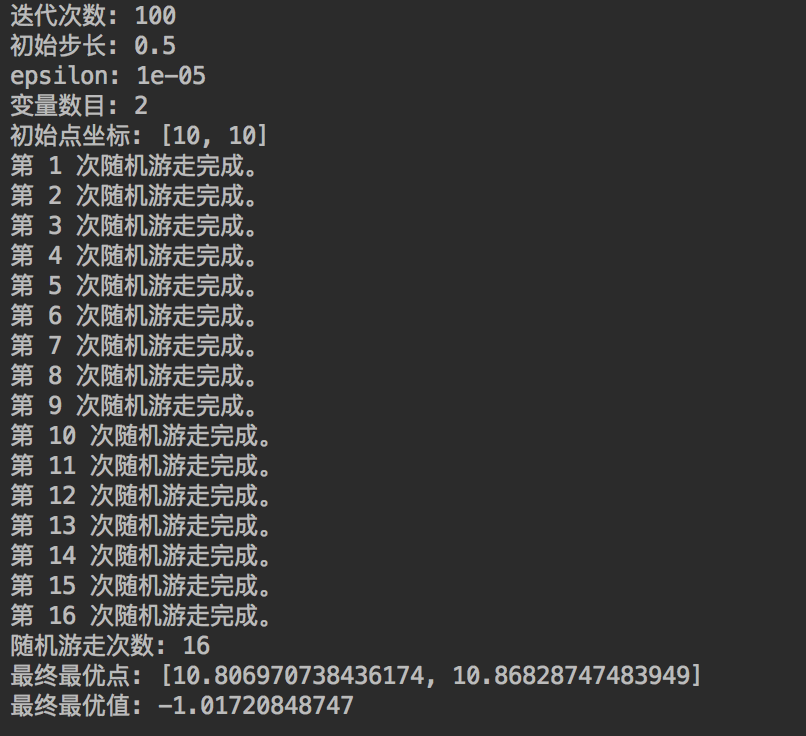### 2. 扩大尝试次数 N、以及步长 λ 有助于脱离局部最优点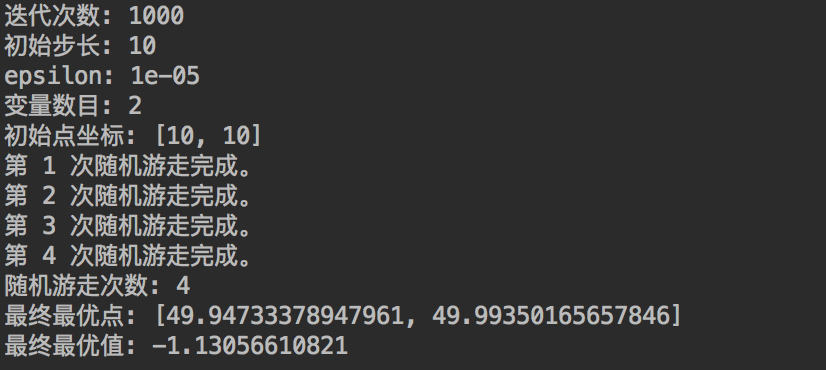## 0x3：改进的随机游走算法

n ui(i=1,2,,n) 标准化得到u1u2un，利用公式 xi = x + λui，min{x1x2xn}替换原来的x1，其他步骤保持不变。

n=10，初始点为(100,10)，N=100λ=10.0ϵ=0.00001，改进的随机游走算法实现代码如下：

#!/usr/bin/env python
# -*- coding: utf-8 -*-

from __future__  import print_function
import math
import random

N = 100     # 迭代次数
step = 10.0     # 初始步长
epsilon = 0.00001
variables = 2   # 变量数目
x = [-100,-10]  # 初始点坐标
walk_num = 1    # 初始化随机游走次数
n = 10  # 每次随机生成向量u的数目

print("迭代次数:", N)
print("初始步长:", step)
print("每次产生随机向量数目:", n)
print("epsilon:", epsilon)
print("变量数目:", variables)
print("初始点坐标:", x)

# 定义目标函数
def obj_function(p):
r = math.sqrt((p-50)**2 + (p-50)**2) + math.e
f = math.sin(r)/r + 1
return -f

# 开始随机游走
while(step > epsilon):
k = 1   # 初始化计数器
while(k < N):
# 产生n个向量u
x1_list = []    # 存放x1的列表
for i in range(n):
u = [random.uniform(-1,1) for i1 in range(variables)]   # 随机向量
# u1 为标准化之后的随机向量
u1 = [u[i3]/math.sqrt(sum([u[i2]**2 for i2 in range(variables)])) for i3 in range(variables)]
x1 = [x[i4] + step*u1[i4] for i4 in range(variables)]
x1_list.append(x1)
f1_list = [obj_function(x1) for x1 in x1_list]
f1_min = min(f1_list)
f1_index = f1_list.index(f1_min)
x11 = x1_list[f1_index]     # 最小f1对应的x1
if(f1_min < obj_function(x)): # 如果找到了更优点
k = 1
x = x11
else:
k += 1
step = step / 2
print("第%d次随机游走完成。" % walk_num)
walk_num += 1

print("随机游走次数:",walk_num-1)
print("最终最优点:",x)
print("最终最优值:", obj_function(x))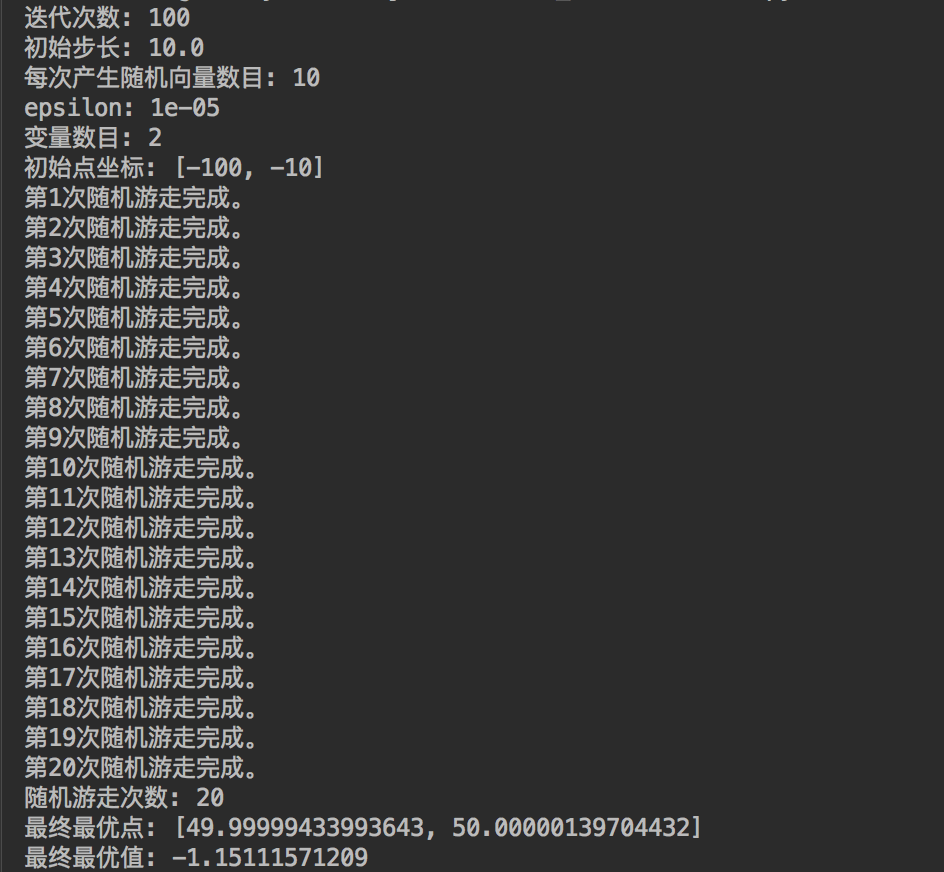## 0x4：步长 λ 的意义

http://www.cnblogs.com/lyrichu/p/7209529.html
http://www.cnblogs.com/lyrichu/p/7209529.html 

# 5. Ramdom Walk Graph Segmentation 随机游走图像分割算法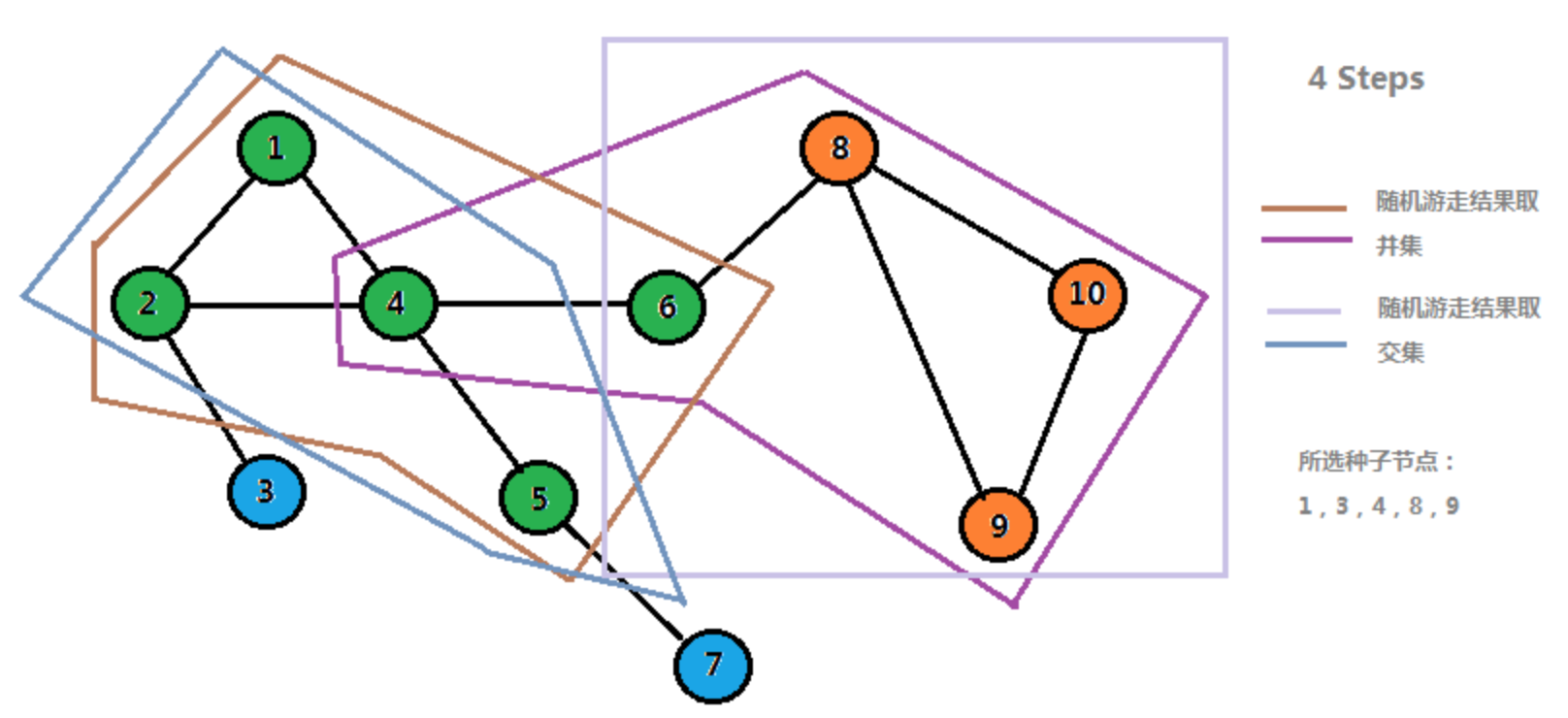## 0x1：基于随机游走的图像分割算法思想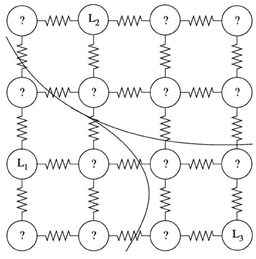## 0x2：算法流程

### 1. 建立图模型

#### 2）边权重矩阵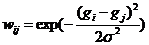，其中，gi 表示个像素点的灰度值、或纹理信息等参数；

#### 3）节点转移概率矩阵

Lij = di：如果 i = j：di为顶点vi的度，是连接顶点的所有边权值之和，di=sumj wij；
Lij = -wij：如果 i j 是相邻节点，wij 是节点i 和节点j 之间的权重；
Lij = 0：other；

### 2. 求解狄利克雷方程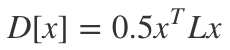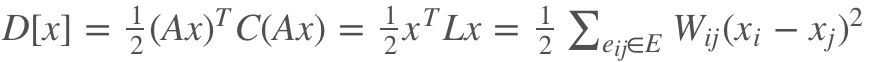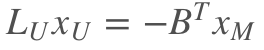### 3. 模型的求解

https://yq.aliyun.com/ziliao/522808
https://blog.csdn.net/Broccoli_Lian/article/details/79739299